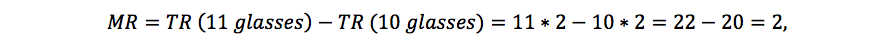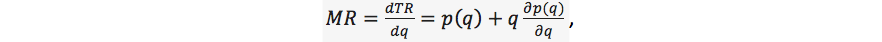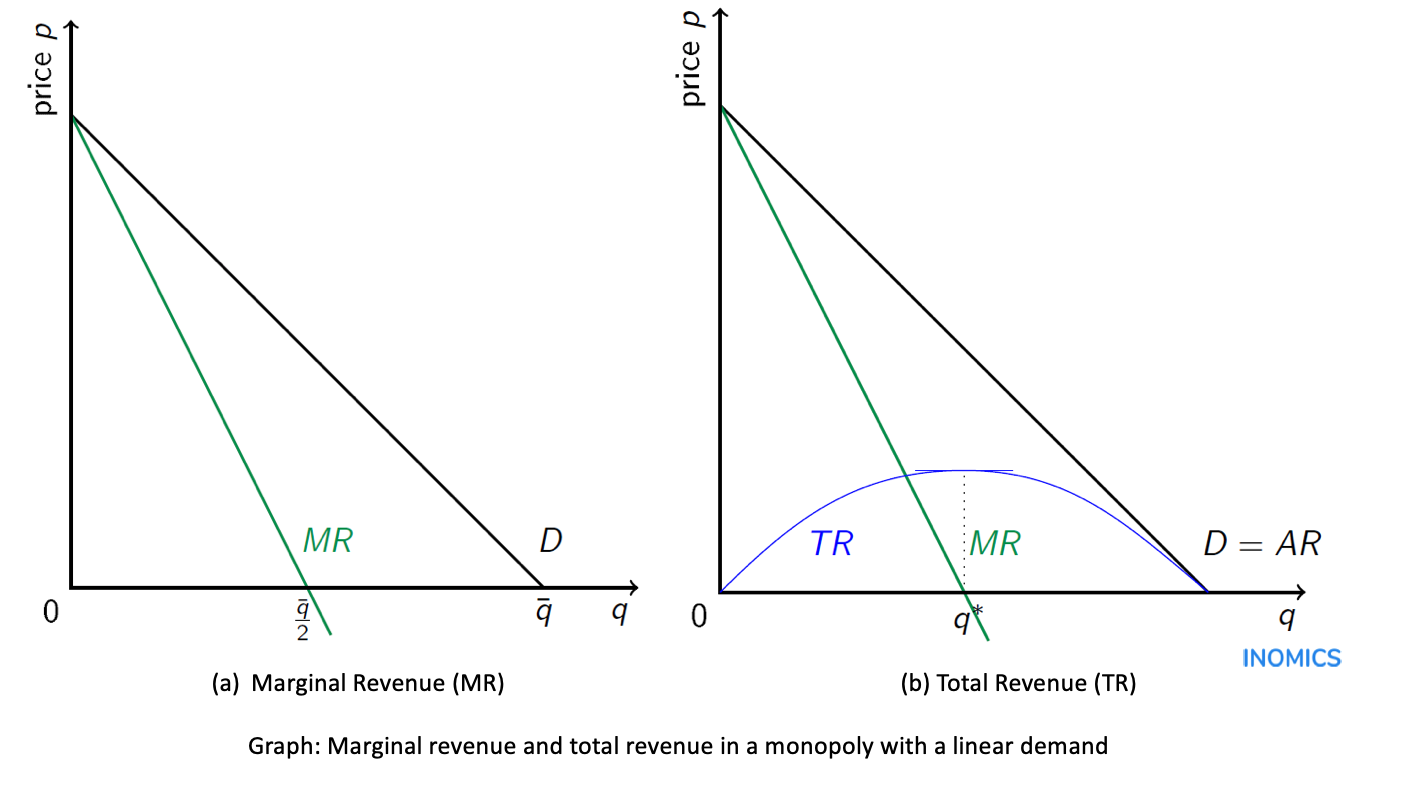# Marginal Revenue

Marginal revenue (MR) measures the change in total revenue that occurs when a firm increases output by one unit, i.e., it is the extra income (or revenue) generated by selling one additional unit.

Whenever a firm increases the number of units it sells this will affect the firm's revenue, because the quantity sold is higher. In a perfectly competitive market, each firm acts as a price taker and can sell as much as it wants to at the market price. This implies that the marginal revenue in a perfectly competitive market is equal to the market price. For example, if a firm is selling orange juice at 2 € per glass (and it can sell as many glasses as it wants to at this price), then any additional glass of orange juice that the firm sells increases total revenue (TR) by 2 and thus, marginal revenue is equal to 2.  That is, if the firm increases quantity say from 10 to 11 glasses, total revenue increases from 20 to 22 and the change in total revenue is equal to 2:which equals the price of the good. If the market is not perfectly competitive, the marginal revenue is not equal to the market price. A monopolist who faces the entire market demand has to lower the price if it wants to sell more. Given that the monopolist has to decrease the price for all units (not only for the additional unit that it wants to sell), total revenue will increase by less than the market price. To be more precise, selling one more unit will increase total revenue by the price at which the good is sold minus the revenue that the monopolist loses, because it have to sell all units at a lower price.

Mathematically the marginal revenue is the derivative of total revenue with respect to quantity, i.e.where TR is total revenue TR p(q)q. The first part of this derivative is positive, because when the quantity sold rises total revenue increases by the price at which the extra unit is sold. The second term of the derivative is negative, because to increase quantity the price per unit has to decrease. Multiplied by the total number of units q, this measures the total loss in revenue due to the lower price.The figure shows the marginal revenue and total revenue for a monopoly with a linear market demand. In the left panel (a) we see the marginal revenue curve and the demand curve. In the case of a linear demand marginal revenue is a linear function as well and its slope is twice as steep as the slope of the demand function. That is, if inverse demand is p a bq, then the marginal revenue is MR 2bq.

The right panel (b) shows the marginal revenue as well as the total revenue (and average revenue) in the case of a linear demand function. Total revenue is a quadratic function which is maximized at the point where marginal revenue is equal to zero (at quantity q*). Keep in mind that marginal revenue is not the same as average revenue AR, which is the revenue per unit, i.e.,Marginal revenue is an important concept in economics, because together with marginal costs it determines the equilibrium quantity and price in a market. A firm maximizes its profits when it produces the quantity at which the marginal revenue of the last unit is equal to marginal costs of this unit. That is, profit maximization requires MR MC.

If marginal revenue exceeds marginal costs (MR MC) this implies that by selling one additional unit the increase in total revenue exceeds the increase in total costs and therefore the profits of the firm will increase if it sells this unit. (Remember that the profit of the firm is total revenue minus total costs). Consequently, a profit maximizing firm will produce this additional unit. If marginal costs exceed marginal revenue (MR MC) total costs increase more than total revenue for this unit and it is better for the firm not to sell this unit. When MR MC the firm has no incentive to neither increase nor decrease its quantity as both would lead to a reduction in profits.

While in general in economics we usually assume that the firms´ objective is to maximize profits, some economists following Baumol (see e.g. “Business Behavior, Economics and Growth”, 1967) argue that under certain conditions firms rather seek to maximize revenue than profits. As main reason Baumol argues that the managers of a firm (in contrast to the owners and shareholders) are more interested in maximizing sales than profits, because there is a direct link between the salary and reputation of a manager and total revenue (sales).

## Good to know

What determines the wage that you can earn? According to economic theory your salary will depend on how much the total revenue of your employer increases by hiring you.  This theory can be used to explain why some football clubs are willing to pay huge sums for players that they expect to contribute considerably to the success of the club. For example, in Summer 2019 Eden Hazard transferred from Chelsea to Real Madrid for €150 million.

## Test whether you understood the concept of marginal revenue...

Take a quiz

Exercises for you